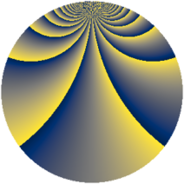# Properties

 Label 1805.2.beLevel $1805$ Weight $2$ Character orbit 1805.be Rep. character $\chi_{1805}(8,\cdot)$ Character field $\Q(\zeta_{228})$ Dimension $13536$ Sturm bound $380$

# Related objects

## Defining parameters

 Level: $$N$$ $$=$$ $$1805 = 5 \cdot 19^{2}$$ Weight: $$k$$ $$=$$ $$2$$ Character orbit: $$[\chi]$$ $$=$$ 1805.be (of order $$228$$ and degree $$72$$) Character conductor: $$\operatorname{cond}(\chi)$$ $$=$$ $$1805$$ Character field: $$\Q(\zeta_{228})$$ Sturm bound: $$380$$

## Dimensions

The following table gives the dimensions of various subspaces of $$M_{2}(1805, [\chi])$$.

Total New Old
Modular forms 13824 13824 0
Cusp forms 13536 13536 0
Eisenstein series 288 288 0

## Trace form

 $$13536 q - 70 q^{2} - 70 q^{3} - 74 q^{5} - 144 q^{6} - 68 q^{7} - 76 q^{8} + O(q^{10})$$ $$13536 q - 70 q^{2} - 70 q^{3} - 74 q^{5} - 144 q^{6} - 68 q^{7} - 76 q^{8} - 70 q^{10} - 144 q^{11} - 76 q^{12} - 70 q^{13} - 52 q^{15} - 524 q^{16} - 80 q^{17} - 76 q^{18} - 92 q^{20} - 164 q^{21} + 108 q^{22} - 70 q^{23} - 66 q^{25} - 152 q^{26} - 76 q^{27} - 58 q^{28} - 104 q^{30} - 152 q^{31} - 40 q^{32} - 228 q^{33} - 104 q^{35} + 256 q^{36} - 76 q^{37} - 126 q^{38} - 4 q^{40} - 104 q^{41} - 34 q^{42} - 222 q^{43} + 130 q^{45} - 228 q^{46} - 98 q^{47} - 124 q^{48} - 228 q^{50} - 60 q^{51} - 58 q^{52} - 88 q^{53} - 72 q^{55} - 380 q^{56} - 124 q^{57} - 8 q^{58} - 190 q^{60} - 188 q^{61} - 42 q^{62} - 116 q^{63} - 228 q^{65} - 68 q^{66} - 94 q^{67} - 60 q^{68} - 94 q^{70} - 140 q^{71} - 208 q^{72} - 36 q^{73} - 76 q^{75} - 76 q^{76} - 60 q^{77} - 160 q^{78} - 98 q^{80} - 500 q^{81} - 108 q^{82} - 88 q^{83} - 42 q^{85} + 1144 q^{86} + 24 q^{87} - 76 q^{88} - 148 q^{90} - 220 q^{91} - 60 q^{92} - 100 q^{93} - 346 q^{95} - 972 q^{96} - 76 q^{97} - 4 q^{98} + O(q^{100})$$

## Decomposition of $$S_{2}^{\mathrm{new}}(1805, [\chi])$$ into newform subspaces

The newforms in this space have not yet been added to the LMFDB.